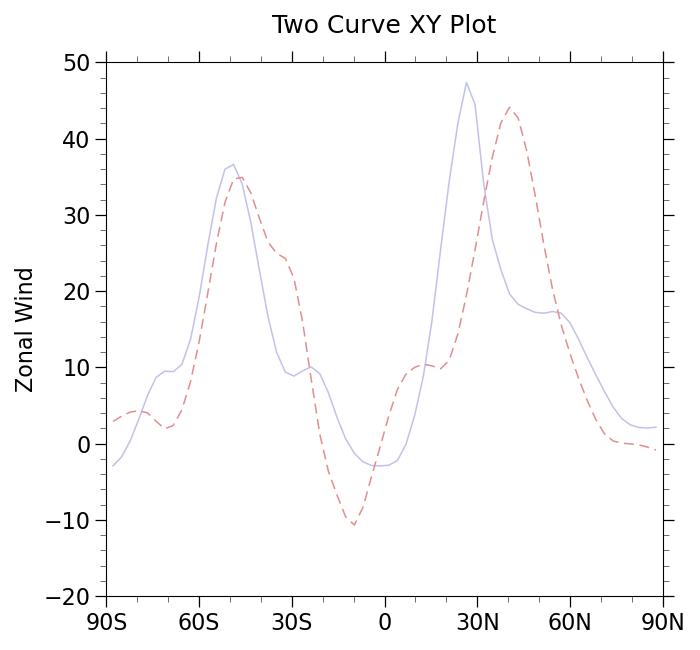# NCL_xy_2_1.py#

This script illustrates the following concepts:
• Drawing an XY plot with multiple curves

• Changing the line color for multiple curves in an XY plot

• Changing the line thickness for multiple curves in an XY plot

• Drawing XY plot curves with both lines and markers

• Changing the default markers in an XY plot

• Making all curves in an XY plot solid.

See following URLs to see the reproduced NCL plot & script:

Import packages:

```import numpy as np
import xarray as xr
import matplotlib.pyplot as plt

import geocat.datafiles as gdf
import geocat.viz as gv
```

```# Open a netCDF data file using xarray default engine and load the data into xarrays
ds = xr.open_dataset(gdf.get("netcdf_files/uv300.nc"))
U = ds.U
```

Plot:

```# Generate figure (set its size (width, height) in inches) and axes
plt.figure(figsize=(7, 6.5))
ax = plt.gca()

# Plot slices of data
U.isel(time=0).sel(lon=82, method='nearest').plot(x="lat",
marker='',
color='#C0C2EA',
linewidth=1.1)
U.isel(time=0).sel(lon=-69, method='nearest').plot(x="lat",
marker='',
color='#E28D90',
linewidth=1.1,
linestyle='--',
dashes=[6.5, 3.7])

# Use geocat.viz.util convenience function to add minor and major tick lines
x_minor_per_major=3,
y_minor_per_major=5,
labelsize=16)

# Use geocat.viz.util convenience function to set axes parameters without calling several matplotlib functions
# Set axes limits, tick values, and tick labels to show latitude & longitude (i.e. North (N) - South (S))
gv.set_axes_limits_and_ticks(
ax,
xlim=(-90, 90),
ylim=(-20, 50),
xticks=np.linspace(-90, 90, 7),
yticks=np.linspace(-20, 50, 8),
xticklabels=['90S', '60S', '30S', '0', '30N', '60N', '90N'])

# Use geocat.viz.util convenience function to set titles and labels without calling several matplotlib functions
gv.set_titles_and_labels(ax,
maintitle="Two Curve XY Plot",
xlabel="",
ylabel="Zonal Wind")

# Show the plot
plt.tight_layout()
plt.show()
```Total running time of the script: ( 0 minutes 0.219 seconds)

Gallery generated by Sphinx-Gallery